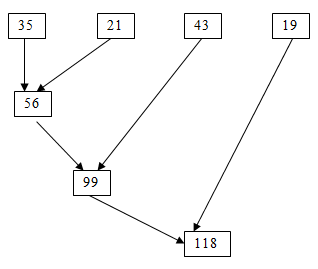# Map, Filter and Reduce Functions in Python

January 24th, 2020

## Introduction

In this tutorial, you are going to learn Map, Filter and Reduce functions in Python. These functions are called as lambda functions or otherwise anonymous functions. These three functions provide a functional approach for programming. These functions are the in-built functions present in python. This type of programming will be expression oriented. Some of the expression oriented functions present in python are:

• Map()
• Filter()
• Reduce()

Let’s have a look at these functions one by one.

### The map() function:

Map() function is a higher-order function that uses another function as a parameter with a sequence of iterables. While applying the function to each of the iterable in the sequence, it returns an output. The syntax for this function is:

 `Map(function to be applied, list of the inputs)`
Generally, we use the list and the sequences with which we perform an operation on each item and we get the result. Say, for example, we update the entire items present in the list by applying for loop:
 ```item_list = [1,2,3,4,5,6] sqrd = [] for a in item_list : sqrd.append(a**2) sqrd [1,4,9,16,25,36]```
We can perform the same operation using the built-in functions present in python. While we use the map(), a function is passed to each item in an iterable object. It then returns a list that contains the results of the entire function call.
 ```item_list = [1,2,3,4,5,6] def sqrt(a); return a**2 list(map(sqrt, item_list))                                     [1,4,9,16,25,36]```
Here, we defined a function and is further applied to every item in the list. i.e., map calls sqrt on every item in the list and then it gets all the return values to a new list. This map() needs a function to be passed in. Generally, we use lambda as the passing function.
 ```List(map((lambda a; a **2), item_list)) [1,4,9,16,25,36]```
As in the above script, lambda function squares every item present in the list.

### The filter() function:

Filter() function is generally used to create an output list that contains values for those functions that return true. The basic syntax of the filter() is:

 `Filter(function defined, iterables)`
Similar to the map(), filter() works in the same way. We can also use user-defined functions and lambda functions in the filter().
 ```def func(a); if a>=5 return a b = filter(func, (1,2,3,4,5,6,7)) print(b) print(list(b))```
Output:
 ``` [5,6,7]```
From the above example, ‘b’ is the filter object and the list contains the list of values which are true for the condition used (a>=5)
We shall use lambda function within the filter() as a parameter which defined the condition that has to be checked. Say for example:
 ```b = filter(lambda a: (a>=5), (1,2,3,4,5,6,7)) print(list(b))```
Output:
 ` [5,6,7]`

### The reduce() function:

As the name implies, reduce function returns only one value for the given function to the iterables. The basic syntax for the reduce() is:

 `reduce(function defined, iterables)`The function actually defines the expression to be applied to the iterable. We can import the function from the functools module. Say for example,
 ```from functools import reduce reduce(lambda x,y; x+y, [35,21,43,19])```
Output:
 ` 118`
As in the example above, reduce() adds each iterable in the list consecutively and finally returns one output alone. It is also possible to use map(), filter() and reduce() functions with one another in python. Let’s see how to do that.

## Applying map(), filter() and reduce() functions with one another:

While using more than one function with one another, initially, the internal function will be solved followed by the outer function.

### Applying filter() along with map():

 ```z = map(lambda b:b+b,filter(lambda b:(b>=5), (1,2,3,4,5,6,7)) print(list(z))```
Output:
 ` [10,12,14]`
While checking the condition ‘b’ greater than equal to 5, we will get [5,6,7] as results. While mapping the condition (b+b), we will get values [10,12,14].

### Applying map() within the filter():

While using the map() within the filter(), we initially operate the iterables as per the condition in a map(). We further check the filter() condition and apply them.

 ```z = filter(lambda b:(b>=5), map(lambda b:b+b, (1,2,3,4,5,6,7)) print(list(z))```
Output:
 ` [8,10,12,14]`

### Applying map() and filter() within the reduce():

As per the condition present in the reduce(), the output of the internal functions will get reduced while using map() and filter() within reduce()

 ```z = reduce(lambda i,j: i+j, map((lambda i:i+i, filter (lambda  i : (i>=3), (1,2,3,4,)) print(z)```
Output:
 ` `
While computing the internal map() and filter() functions, we will get [6,8] as the output.
The tutorial ends with this. Hope you understand the use of map(), filter() and reduce() functions in python. See you in the next tutorial.

Social Share: